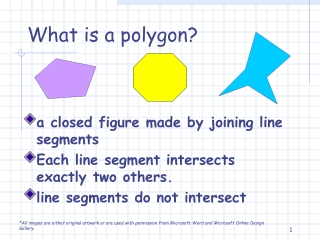DownloadDownload PresentationWhat is a polygon?

# What is a polygon?

Download Presentation## What is a polygon?

- - - - - - - - - - - - - - - - - - - - - - - - - - - E N D - - - - - - - - - - - - - - - - - - - - - - - - - - -
##### Presentation Transcript

1. a closed figure made by joining line segments Each line segment intersects exactly two others. line segments do not intersect What is a polygon? *All images are either original artwork or are used with permission from Microsoft Word and Microsoft Online Design Gallery.

2. Are these figures polygons? yes no yes no

3. A regular polygon is a polygon whose sides are all the same length and whose angles are all the same. What is a regular polygon?

4. The sides are not all the same length. The angles are not all the same. These are not examples of regular polygons.

5. triangle What is a three-sided polygon? Let’s look at some different kinds of polygons.

6. parallelogram What is a four-sided figure whose opposite sides are parallel? Polygons

7. rectangle What is a four-sided polygon whose sides and angles are all the same? Polygons

8. square What is a parallelogram or rectangle with four equal sides and angles? Polygons

9. rhombus What is a parallelogram whose sides are all the same length? Polygons

10. trapezoid What is a four-sided polygon having exactly one pair of parallel lines? Polygons

11. pentagon What is a five-sided polygon? Polygons

12. hexagon What is a six-sided pentagon? Polygons

13. heptagon What is a seven-sided pentagon? Polygons

14. octagon What is an eight-sided pentagon? Polygons

15. regular polygon triangle parallelogram rectangle square rhombus trapezoid pentagon hexagon heptagon octagon Let’s review the polygons we‘ve learned about today.

16. Can you identify these polygons?

17. Using your geoboards or dot paper, replicate these polygons.                                